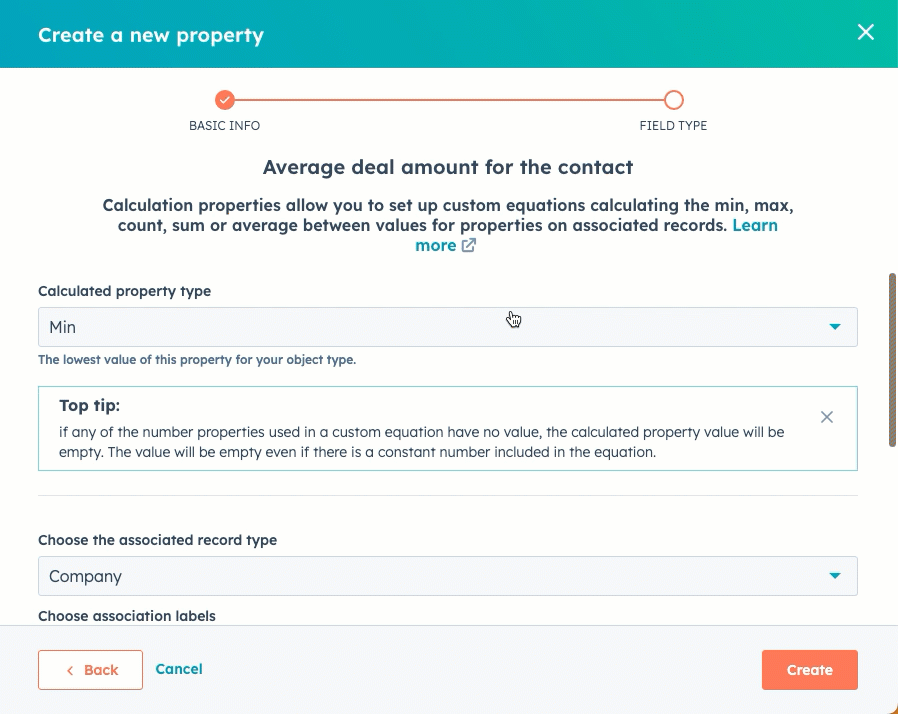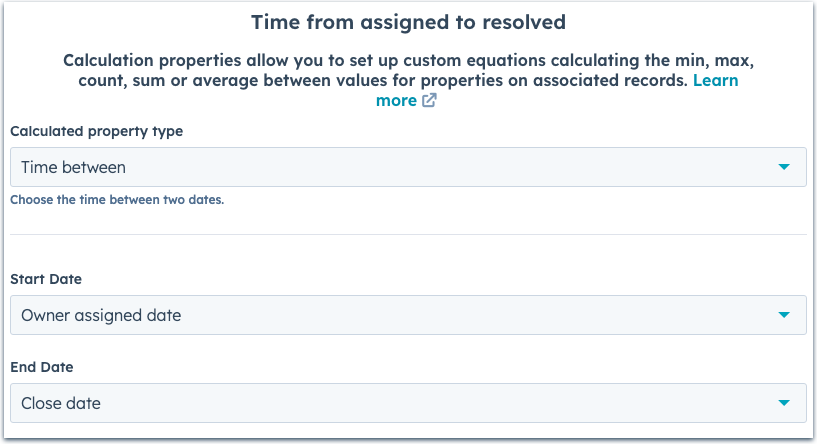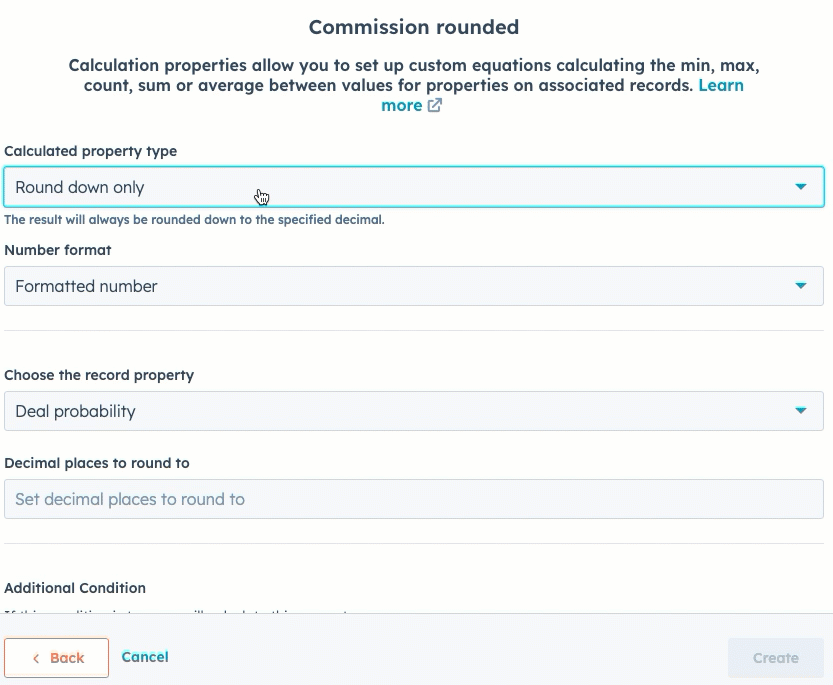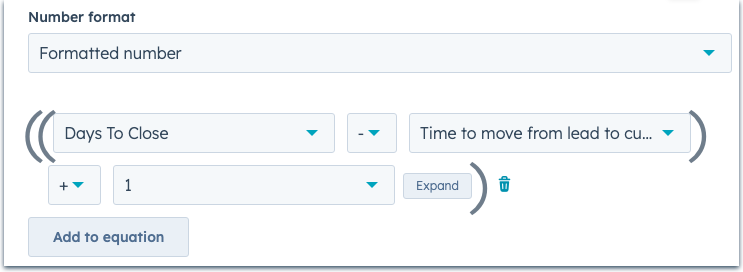#### Available with any of the following subscriptions, except where noted:Marketing Hub Professional, EnterpriseSales Hub Professional, EnterpriseService Hub Professional, EnterpriseOperations Hub ProfessionalCMS Hub Professional, Enterprise

Calculation properties in HubSpot allow you to automatically calculate the min, max, count, sum, or average value for properties on associated records or set up custom equations based on other properties.

For example, you can create:

• A contact property that calculates the average Amount of a contact's associated deals.
• A company property that calculates the cost to acquire a new customer based on a custom equation.

Please note: depending on your HubSpot subscription, there is a limit to the number of calculation properties you can create.

## Create calculation properties

Calculation properties can be set up based on different criteria, including property values of associated records, the time between two properties' values, or your own custom equations. To create a calculation property:

• In your HubSpot account, click the settings settings icon in the main navigation bar.
• In the left sidebar menu, navigate to Properties.

• Click the Select an object dropdown menu, then select [Object] properties to create a property for that object.

• Enter the basic information for your property, then click Next.
• Select Calculation for the Field type. Once your Calculation property is created, the field type cannot be edited.
• Click Build calculation.
• Click the Calculated property type dropdown menu and select the type of formula you want to create:

### Calculate values based on properties of associated records

You can create properties for an object that will be calculated based on certain property values of that object's associated records. You can calculate based on all associated records of that object or for specific association labels, including parent/child company relationships.

For example, you can create a deal property that counts the total number of sales activities logged for a deal's associated contacts with the Decision maker label.

• Click the Calculated property type dropdown menu and select on of the following types:
• Min: displays the minimum value for the selected number property on associated records of the selected object.
• Max: displays the maximum value for the selected number property on all associated records of the selected object.
• Count: counts the total number of associated records of the selected object that have a value for the number property selected.
• Sum: sums up the values for the selected number property on all associated records of the selected object.
• Average: calculates the average of the values for the selected number property on all associated records of the selected object.
• To choose how to format the property's values, click the Number format dropdown menu, then select from the following formats: Formatted Number, Unformatted number, Currency, Percentage, or Duration.

Please note: all calculation properties using Currency as the number format will use your account's Company currency• To add an additional condition, click Create condition. When an additional condition is set up, that condition must be met in order for the property to be calculated (e.g., calculate the property when Recent deal amount > 1000).
• In the first dropdown menu, select a property.
• In the second dropdown menu, select an operator that will compare the first property's value to a number or to another property's value.
• In the third dropdown menu, select a property or enter a number that will be compared to the first property.
• Click Create. Once created, the property's displayed field type will be Rollup.

### Calculate values based on time between two properties

You can create properties that calculate the time between two selected date picker properties within the same object. For example, you can calculate the time between when a ticket's owner was assigned and when the ticket was closed. Learn more about how Time Between calculation property values are displayed.

• Click the Calculated property type dropdown menu and select Time between.
• Click the dropdown menus to select your Start date and End date properties.• To add an additional condition, click Create condition. When an additional condition is set up, that condition must be met in order for the property to be calculated (e.g., calculate the property when Recent deal amount > 1000).
• In the first dropdown menu, select a property.
• In the second dropdown menu, select an operator that will compare the first property's value to a number or to another property's value.
• In the third dropdown menu, select a property or enter a number that will be compared to the first property.
• Click Create. Once created, the property's displayed field type will be Rollup.

The following behavior is expected when displaying values for time between calculation properties:

• The time will be displayed differently in different HubSpot tools.
• For date properties without a timestamp, the default time used in the calculation will be 12:00 am UTC for the selected day.
• In an object record (e.g., a contact record), the displayed value will be in hours, days, months, or years, and will be rounded up to the next unit based on specific thresholds. For example, if the time calculated is one month and twenty days, then the value displayed will be One month, but if the time calculated is one month and twenty-seven days, then the value displayed will be Two months. The thresholds are:
• 45 seconds or more rounds up to one minute
• 45 minutes or more rounds up to one hour
• 22 hours or more rounds up to one day
• 26 days or more rounds up to one month
• 11 months or more rounds up to one year
• In custom reports that use a property with this field type, the displayed value will be in days. For example, if the time calculated is one year and twenty days, then the value displayed will be 385 days.

Please note: within HubSpot, the raw values of Time between and Average property types are stored in milliseconds. If an equation with these values yields a higher value than expected, use a millisecond converter to convert to your desired unit of time.

### Calculate values based on rounding of property values

You can create calculation properties that specify how to round number values of other properties. For example, you can create a calculation property that rounds a commission property up to the nearest two decimal places.

• Click the Calculated property type dropdown menu and select a rounding option:
• Round down only: always round values down to the specified number of decimal places.
• Round up only: always round values up to the specified number of decimal places.
• Round to nearest: round values to the nearest specified number of decimal places based on proximity.
• To choose how to format the property's values, click the Number format dropdown menu, then select from the following formats: Formatted Number, Unformatted number, Currency, Percentage, or Duration.
• Click the Choose the record property dropdown menu, then select the property with the values you want to round. You can select any score, calculation, or number property within that object.
• In the Decimal places to round to text box, enter the number of decimal spaces you want the values rounded to.

Please note: for currency properties, this feature will round the value to the precision chosen, but will still display values as a currency with the correct amount of digits.• To add an additional condition, click Create condition. When an additional condition is set up, that condition must be met in order for the property to be calculated (e.g., calculate the property when Recent deal amount > 1000).
• In the first dropdown menu, select a property.
• In the second dropdown menu, select an operator that will compare the first property's value to a number or to another property's value.
• In the third dropdown menu, select a property or enter a number that will be compared to the first property.
• Click Create. Once created, the property's displayed field type will be Calculation.

### Calculate values based on a custom equation

You can also create properties that calculate values based on your own criteria. Custom equations can only be set up for number properties within the same object for which you're creating the new property. For example, you can create an equation to calculate the amount from a company's most recent deal (Most recent deal amount) compared to how many times your business contacted the company (Number of times contacted).

To build your equation, you can use the dropdown menus with pre-defined options, or use the advanced mode editor to write equations with arithmetic operators, comparison operators, logic operators, conditional statements, and other functions. Learn more about the available operators and functions, as well as how to format your formulas.

• Click the Calculated property type dropdown menu, then select Custom equation.
• To create an equation with the dropdown menu options:
• Click the Number format dropdown menu, then select how to format the property's values. You can select one of the following: Formatted Number, Unformatted number, Currency, Percentage, or Duration.
• Click a dropdown menu to select a number property, or type a number and click the Constant: [entered value] option to include a constant integer in your equation.
• Click the function dropdown menu to add (+), subtract (-), multiply (*), or divide (/).• To create an equation in the advanced mode editor:
• Click to toggle the Advanced mode switch on.• Click the Output type dropdown, then select the type of output your calculation will return. You can select one of the following: Number, Boolean, String, or Date
• If you've selected the Number output type, click the Number format dropdown menu, then select how to format the property's values. You can select one of the following: Formatted Number, Unformatted number, Currency, Percentage, or Duration.
• In the editor, insert, type, or paste functions and properties to build your custom equation. Learn more about custom calculation equation syntax. To insert formula elements:
• Click the Insert dropdown menu.
• To insert functions into your equation, select Functions, then select a function.
• To insert properties into your equation, select Properties, then select a property. You can only create custom equations using properties of the same object for which you're creating the property.• If your equation has errors, click [x] issues above the editor to view the reasons for the errors. You'll be unable to create the property if your equation has unresolved errors. Learn more about how to format your equations.

Please note: if any of the number properties in a custom equation have no value, or a null value, the calculation will not run and the calculation property value will be empty. The value will be empty even if there is a constant number included in the equation.

• To add an additional condition, click Create condition. When an additional condition is set up, that condition must be met in order for the property to be calculated (e.g., calculate the property when Recent deal amount > 1000).
• In the first dropdown menu, select a property.
• In the second dropdown menu, select an operator that will compare the first property's value to a number or to another property's value.
• In the third dropdown menu, select a property or enter a number that will be compared to the first property.
• Click Create. Once created, the property's displayed field type will be Calculation.

## Custom equation syntax

In the advanced mode, you can build your own custom equations using literal data, data from other properties, operators, and functions. Below, learn about the syntax for writing custom calculation equations.

### Literal syntax

Using literal data, you can add strings of text, numbers, and true or false values to your equations. You can format strings, numbers, and boolean constants in the following ways:

• String literal: text surrounded by quotation marks. These can be represented with either single quotes (`'constant'`) or double quotes (`"constant"`).

• Number literal: numbers without quotation marks. Constant numbers can be any real numbers, and can include point notation. For example, both `1005` and `1.5589` are valid constant numbers.

• Boolean literal: constant booleans can be `true` or `false`.

### Property syntax

You can include other properties of the same object in your custom equation. If a property is inserted into the editor, it is automatically formatted and validated as part of an equation.

If you choose to manually type or paste a property into the editor, it should be formatted as `[properties.{propertyInternalName}]`. For example, to add the property Test score, which has an internal value of Test_score, you'd type it into the editor as `[properties.Test_score]`.

### Operators

You can use operators in your equation to update, evaluate, or compare constants and property values. When using multiple operators, they follow the PEMDAS order of operations. Depending on the type of data you're using, you can include the following operators in your custom equations.

 Operator Description `+` Add numbers or strings. Returns a number. `-` Subtract numbers. Returns a number. `*` Multiply numbers. Returns a number. `/` Divide numbers. Returns a number. `<` Checks if a value is less than another. Supported by number properties or constants. Returns a boolean. `>` Checks if a value is greater than another. Supported by number properties or constants. Returns a boolean. `<=` Checks if a value is less than or equal to another. Supported by number properties or constants. Returns a boolean. `>=` Checks if a value is greater than or equal to another. Supported by number properties or constants. Returns a boolean. `!` Checks if none of the values are true. Returns a boolean. `=` or `==` Checks if a value is equal to another. Supported by numbers and strings. Returns a boolean. `!=` Checks if a value is not equal to another. Supported by numbers and strings. Returns a boolean. `or` or `||` Checks if either of two values are true. Returns a boolean. `and` or `&&` Checks if both values are true. Returns a boolean.

### Functions

You can use functions within custom formulas to calculate values and complete actions with your data. Depending on the type of data you're using, you can include the following functions in your custom equations.

 Function Arguments Examples `concat` Combine strings into one value. Returns a string. `concat("arg 1", "arg 2")` arg1: the string to which arg2 will be appended. arg2: the string to append to arg 1. `concat("Test", " example")` = "Test example" `currency_decimal_places` Get the number of decimal places for a currency. Returns a number. `currency_decimal_places(currency)` currency: the currency string for which you want to calculate the number of decimal places. `currency_decimal_places("USD")` `dated_exchange_rate` Get the value of an exchange rate at the given time. Returns a number. `dated_exchange_rate(currency, date)` currency: the currency string for which you want to calculate the exchange rate on a specific date (e.g., `"USD"`). date: the datetime property for which you want to calculate the exchange rate. `dated_exchange_rate("USD", [properties.closedate])` `exchange_rate` Get the most recent exchange rate. Returns a number. `exchange_rate(currency)` currency: the currency string for which you want to calculate the exchange rate. `exchange_rate("USD")` `if` Set up a conditional expression. `if(condition, arg2)` or `if(condition, arg2, arg3)` condition: the boolean statement that determines what the calculated value will be. arg2: a boolean, number, or string that will be the calculated value if the condition is met.  arg3 (optional): the calculated value if the condition is not met. If included, it must be the same type as `arg2`. If not used, the value will be `null` when the condition is not met. `if([properties.amount] > 1000, "large", "small")` `is_present` Determine if a value is present for the token. Returns a boolean. `is_present(property)` property: the property you want to check for a value. `is_present([properties.name])` = true `last_updated` Get the date of when the token was last updated. Returns a timestamp in milliseconds as a string. `last_updated(property)` property: the property for which you want to check the most recent update date. `last_updated([properties.amount])` = "1672531200000" `lowercase` Change all characters to lowercase. Returns a string. `lowercase(text)` text: the string or text property with values you want to change to lowercase. `lowercase("heLLo")` = "hello" `lowercase([properties.test])` `max` Get the maximum of two numbers. Returns a number. `max(arg1, arg2)` arg1: the first number or number property. arg2: the second number or number property. `max(500,[properties.amount])` = 500, if the amount value is less than 500. `min` Get the minimum of two numbers. Returns a number. `min(arg1, arg2)` arg1: the first number or number property. arg2: the second number or number property. `min(500, 250)` = 250 `month` Get the numeric month of a given date. Returns a number. `month(date)` date: the datetime property for which you want to calculate the month. `month([properties.closedate])` `number_to_string` Convert a number to a string. Returns a string. `number_to_string(number_to_convert)` number_to_convert: the number or number property you want to change to a string. `number_to_string(1000)` = "1000" `pipeline_probability` Get the probability of a pipeline stage. Returns a number. `pipeline_probability(pipeline)` pipeline: the name of the pipeline for which you want to calculate the probability. `pipeline_probability("newdeal")` `round_down` Round a number down to a specified precision. Returns a number. `round_down(number_to_round, precision)` number_to_round: the number or number property you want to round down. precision: the number of decimal places to round down to. `round_down(4.353, 1)` = 4.3 `round_nearest` Round a number to a specified precision. Returns a number. `round_nearest(number_to_round)` number_to_round: the number or number property whose values you want to round to the nearest whole number. `round_nearest(4,353)` = 4 `round_up` Round a number up to a specified precision. Returns a number. `round_up(number_to_round, precision)` number_to_round: the number or number property whose values you want to round up. precision: the number of decimal places to round up to. `round_up(4.353, 1)` = 4.4 `string_to_number` Convert a string to a number. Returns a number. `string_to_number(string_to_convert)` string_to_convert: the string or text property whose values you want to convert to a number. `string_to_number("1000")` = 1000 `time_between` Get the time difference between two dates. Returns a number. `time_between(date1, date2)` date1: the starting datetime property. date2: the end datetime property. `time_between([properties.createdate],[properties.closedate])` `uppercase` Change all characters to uppercase. Returns a number. `uppercase(text)` text: the string or text property you want to change to uppercase. `uppercase("hello")` = "HELLO" `year` Get the numeric year of a given date. Returns a number. `year(date)` date: the datetime property for which you want to calculate the year. `year([properties.closedate])`